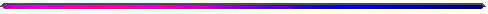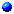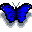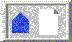##Courses in Dynamical Systems At Boston University###MA 226 Ordinary Differential Equations

A sophomore level differential equations course taught from a modern perspective using ideas from dynamical systems theory. This course has been developed at Boston Univesity as part of the BU Differential Equations Project. Prerequisites: calculus. Offered each term.

###MA 471/671 Chaotic Dynamical SystemsA course in discrete dynamical systems taught at the sophomore-junior level. MA 671 is available for graduate credit for students from outside of the Mathematics Department. Prerequisites: calculus. Offered most years in Fall.

###MA 561 Methods of Applied Math I

This is an introduction to PDEs, taught at the advanced undergraduate/beginning graduate level. Topics can vary by instructor, but it typically covers the derivation and analysis of the classical equations of mathematical physics: heat equation, wave equation, and potential equation; initial and boundary value problems; the method of separation of variables; eigenvalue problems; eigenfunction expansions; and Fourier analysis. It is typically offered each fall. Prerequisites: MA 226 or MA 231.

###MA 562 Methods of Applied Math II

This course covers further topics in PDEs, building on MA 561. Topics vary by instructor, but have included topics such as calculus of variations, first-order non-linear partial differential equations, Hamilton-Jacobi theory, Rayleigh-Ritz procedure, and perturbation methods. It is typically offered each spring. Prerequisites: MA 561.

###MA 565 Mathematical Models in the Life Sciences

An introduction to mathematical modeling, using applications in the biological sciences. Mathematics includes linear difference and differential equations, and an introduction to nonlinear phenomena and qualitative methods. An elementary knowledge of differential equations and linear algebra is assumed. Prerequisites: MA 226 or MA 231.

###MA 573 Qualitative Theory of Ordinary Differential Equations

A course in continuous time dynamical systems, taught at the advanced undergraduate/beginning graduate level. It typically covers topics such as eigenvalues, eigenvectors, Jordan normal forms. Linear systems of differential equations, Phase portrait, Hamiltonian systems, stability theory. Applications to systems arising in mechanics, economics, ecology, electrical circuit theory, etc. It is typically offered in the fall. Prerequisites: MA 226 or MA 231, and MA 242 or MA 442.

###MA 579 Numerical Methods for Biological Sciences

Introduction to the use of numerical methods for studying mathematical models of biological systems. Emphasis on the development of these methods; understanding their accuracy, performance, and stability; and their application to the study of biological systems. Prerequisites: MA 226 or MA 231, and elementary knowledge of linear algebra.

###MA 771 Discrete Dynamical Systems

A course in discrete dynamical systems taught at the graduate level. Topics typically include diffeomorphisms and flows; periodic points, nonwandering points, and recurrent points; hyperbolicity, topological conjugacy, and structural stability; stable manifold theorem; symbolic dynamics; Axiom A and chaotic systems. Prerequisites: linear algebra, real analysis.

###MA 775 Ordinary Differential Equations

A advanced course in continuous time dynamical systems taught at the beginning graduate level. Topics typically include stable and center manifolds theorem, linearization of vector fields, variational equations, Floquet theory and Poincare; maps for periodic orbits, bifurcation of rest points, averaging theory, topics from singular perturbations, Hamiltonian systems, non-linear oscillations, normal forms, and applications. Prerequisites: linear algebra, real analysis.

###MA 776 Partial Differential Equations

An introduction to PDEs taught at the beginning graduate level. Topics typically include linear hyperbolic, elliptic, and parabolic equations; conservation laws and the method of characteristics, Sobolev spaces, and Fourier techniques. Prerequisites: MA 711 (graduate real analysis).

###MA 871 and MA 872 Seminars in Dynamical Systems

This course covers advanced topics in dynamical systems. Topics vary from term to term and are typically choses based on the interests of the students and the faculty member who is teaching the course in any given semester.

###MA 876 Seminar in Partial Differential Equations

This course covers advanced topics in partial differential equations. Topics vary from term to term and are typically choses based on the interests of the students and the faculty member who is teaching the course in any given semester.

There are several other courses that may be of interest, such as MA 777, Multiscale Analysis, and MA 861, Numerical Methods for PDEs, which will become a regularly offerred 700-level course soon. Note that math graduate students can get course credit for any course numbered 500 or higher (except MA 671). Prerequisites are strong suggestions; alternatively students may obtain the consent of the instructor to take a course. For a complete list of math department course information, including current semester course offerings, please go here.BU Dynamical Systems Home PageBU Math Home Page